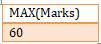# SQL FUNCTIONS

SQL Functions | SQL Tutorial | Minigranth

# SQL Functions : Introduction

• SQL functions are special classical functions that are used to perform specific tasks when combined in with SQL queries. They are basically arithmetic functions.
• They are used in tables with columns having numerical values. There exists five SQL functions which are majorly used and all of them are listed and explained below.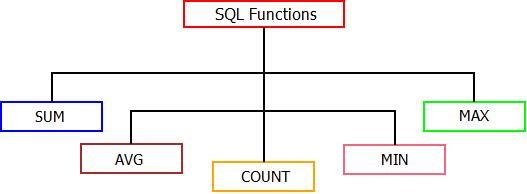SQL Functions : Types

### SQL Functions : SUM

• The “SUM” function as the name suggests, is used to calculate sum for a particular column. Below is the syntax and example.
 Syntax : SELECT SUM(Column_Name) From Table_Name Where “Condition”;

Example : Consider the student_details table. We can find the total sum of marks of students by using below query.

Table : student_details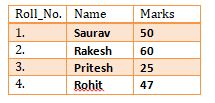Query : SELECT SUM(Marks) From student_details;

Output :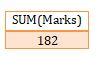### SQL Functions : AVG

• The AVG (Average) function as the name suggests is used to calculate average for a particular column containing numeric values.
 Syntax : SELECT AVG(Column_Name) From Table_Name Where “Condition”;

Example : Consider the student_details table. We can calculate the average of marks for the students using “AVG” function using below query.

Table : student_detailsQuery : SELECT AVG(Marks) From student_details table;

Output :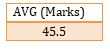### SQL Functions : COUNT

• The "COUNT" function calculates the number of rows that exists in a column which follows a particular condition.
 Syntax : SELECT COUNT(Column_Name) From Table_Name Where “Condition”;

Example : Consider the student_details table. If we want to “COUNT” the total number of students present in the table, it can be done using below query.

Table : student_detailsQuery : SELECT COUNT(Roll_No) From student_details;

Output :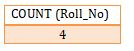### SQL Functions : MIN

• The SQL “MIN” function is used to return smallest possible value from a particular column containing numeric values.
 Syntax : SELECT MIN(Column_Name) From Table_Name Where “Condition”;

Example : Consider the student_details table. If we want to select the minimum marks of a student, it can be done by executing below query.

Table : student_detailsQuery : SELECT MIN(Marks) From student_details;

Output :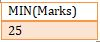### SQL Functions : MAX

• The SQL “MAX” function is used to return largest possible value from a particular column containing numeric values.
 Syntax : SELECT MAX(Column_Name) From Table_Name Where “Condition”;

Example :  Consider the student_details table. If we want to select the maximum marks of a student, it can be done by executing below query.

Table : student_detailsQuery : SELECT MAX(Marks) From student_details;

Output :